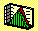2.10 SUMMARY OF PORTFOLIO STATISTICS

The return and risk of a portfolio are measured by calculating the portfolio mean (or expected return) and the portfolio volatility (or standard deviation). Operationally, these statistics are defined as follows.

Given a portfolio a, the expected return of the portfolio is: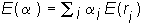if using sample means, the portfolio mean is: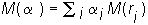Similarly, the portfolio variance is well defined for both the population variance,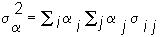and the sample variance: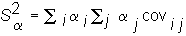Finally, the portfolio's volatility or standard deviation is defined as the square root of the portfolio variance.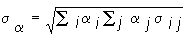and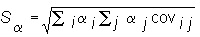In topic 2.11 we apply these concepts to two examples.

previous topic

next topic